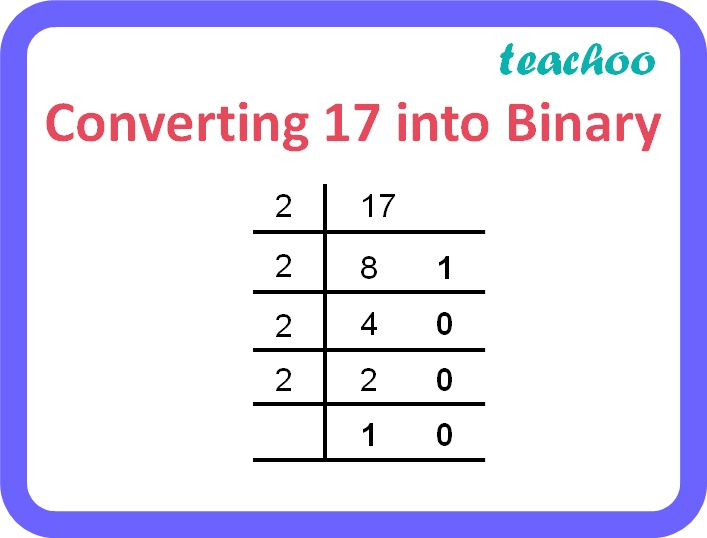3 Mark Questions

Computer Science - Class 11
Chapter 2 Class 11 - Encoding Schemes and Number System

## Convert (17.35)10 to binary form.

17.35 10 is a decimal number.

17 is the integer part of the number and 35 is the fractional part of the number.

Step 1: Converting 17 to binarySo equivalent binary of integer part of the decimal number is 10001 2

Step 2: Converting .35 to binary

 Integer Part Fractional Part 0.35 x 2 = 0.70 0 ↓ 0.70 0.70 x 2 = 1.40 1  ↓ 0.40 0.40 x 2 = 0.80 0  ↓ 0.80 0.80 x 2 = 1.60 1  ↓ 0.60 0.60 x 2 = 1.20 1  ↓ 0.20 0.20 x 2 = 0.40 0  ↓ 0.40

So equivalent binary of fractional part of the decimal number is (. 010110) 2

Therefore, (17.35) 10 = ( 10001.010110) 2

Learn in your speed, with individual attention - Teachoo Maths 1-on-1 Class

### Transcript

Converting 17 into Binary